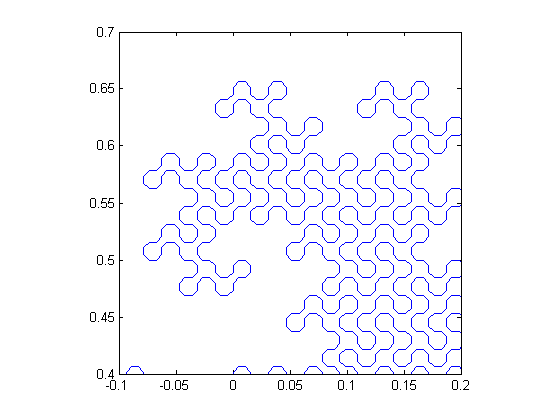# Generating Hilbert curves

This week I came across some files I wrote about 16 years ago to compute Hilbert curves. A Hilbert curve is a type of fractal curve; here is a sample: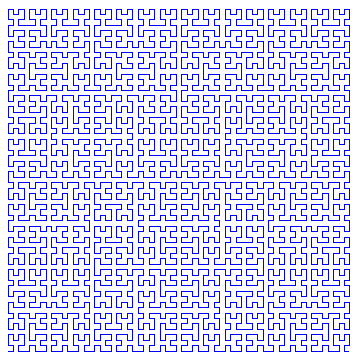I can't remember why I was working on this. Possibly I was anticipating that 16 years in the future, during an unusually mild New England winter, I would be looking for a blog topic.

Anyway, there are several interesting ways to code up a Hilbert curve generator. My old code for generating the Hilbert curve followed the J. G. Griffiths, "Table-driven Algorithms for Generating Space-Filling Curves," Computer-Aided Design, v.17, pp. 37-41, 1985. Here's the basic procedure:

First, initialize four curves, $A_0$, $B_0$, $C_0$, and $D_0$, to be empty (no points).

Then, build up the Hilbert curve iteratively as follows:

$A_{n+1} = [B_n, N, A_n, E, A_n, S, C_n]$

$B_{n+1} = [A_n, E, B_n, N, B_n, W, D_n]$

$C_{n+1} = [D_n, W, C_n, S, C_n, E, A_n]$

$D_{n+1} = [C_n, S, D_n, W, D_n, N, B_n]$

where $N$ represents a unit step up, $E$ is a unit step to the right, $S$, is a unit step down, and $W$ is a unit step left.

One way to code this procedure is to incrementally build up a set of vectors that define the step from one point on the path to the next, and then to use a cumulative summation at the end to turn the steps into x-y coordinates. Here's how you might do it.

A = zeros(0,2);
B = zeros(0,2);
C = zeros(0,2);
D = zeros(0,2);

north = [ 0  1];
east  = [ 1  0];
south = [ 0 -1];
west  = [-1  0];

order = 3;
for n = 1:order
AA = [B ; north ; A ; east  ; A ; south ; C];
BB = [A ; east  ; B ; north ; B ; west  ; D];
CC = [D ; west  ; C ; south ; C ; east  ; A];
DD = [C ; south ; D ; west  ; D ; north ; B];

A = AA;
B = BB;
C = CC;
D = DD;
end

A = [0 0; cumsum(A)];

plot(A(:,1), A(:,2), 'clipping', 'off')
axis equal, axis off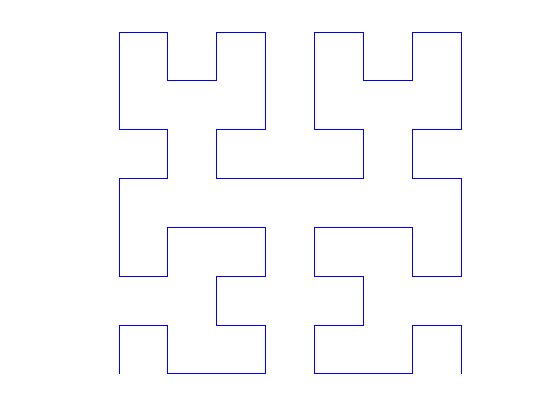I was curious to see what might be on the MATLAB Central File Exchange, so I searched for "hilbert curve" and found several interesting contributions. There are a couple of 3-D Hilbert curve generators, and several different ways of coding up a 2-D Hilbert curve generator. I was particularly interested in the Fractal Curves contribution by Jonas Lundgren.

Jonas shows a much more compact implementation of the ideas above using complex arithmetic. It looks like this:

a = 1 + 1i;
b = 1 - 1i;

% Generate point sequence
z = 0;
order = 5;
for k = 1:order
w = 1i*conj(z);
z = [w-a; z-b; z+a; b-w]/2;
end

plot(z, 'clipping', 'off')
axis equal, axis off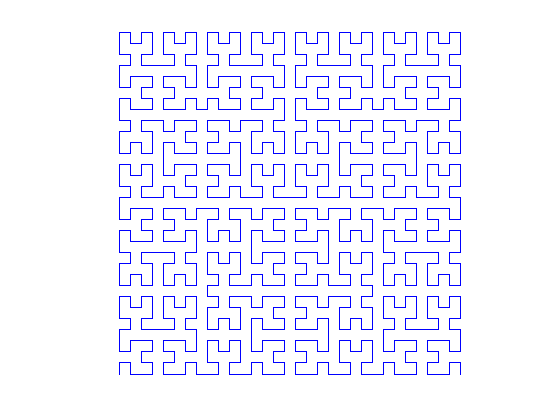Jonas' contribution includes several other curve generators. Here's one called "dragon".

z = dragon(12);
plot(z), axis equal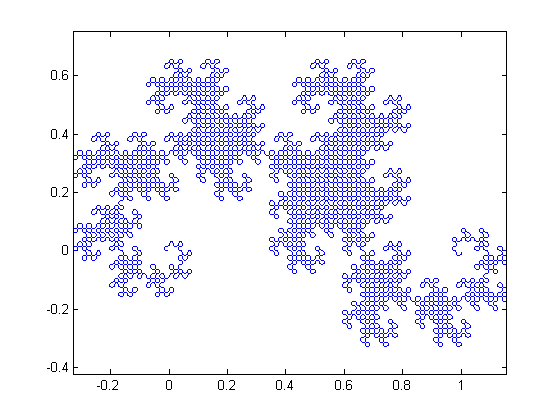Here's a zoom-in view of a portion of the dragon.

axis([-.1 0.2 0.4 0.7])